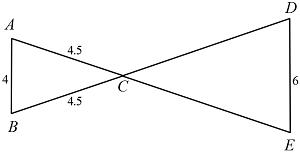# SAT Congruence and SimilarityIn the figure above, $\overline{AB} \parallel \overline{DE}$ and $\overline{AE}$ intersects $\overline{BD}$ at point $C.$ What is the perimeter of $\triangle CED?$

(A) $\ \ 6.75$
(B) $\ \ 12$
(C) $\ \ 13$
(D) $\ \ 19.5$
(E) $\ \ 22.5$

×